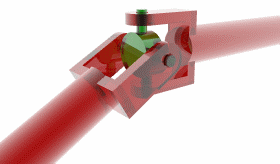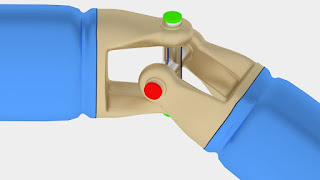# What is universal joint and how it works.Image credit: Google image

Universal Joints are commonly used to transfer mechanical power between two shafts when, their axes are at an angle to each other.

their invention dates back many centuries, Even though the universal joints mechanism seems simple the physics behind this.

This mechanism are rather complicated and interesting.

The universal Joint has three basic parts,

two yolks.
a cross.Image credit : Google image

Now let's consider different power transmitting scenarios,

### In the first case

the input and output shaft are connected in a straight line.
in this case motion is really simple The input Shaft will turn the cross,

And the cross will turn the output shaft it is clear that both the input, and output shafts will turn at the same speed.

Now let's see

### what happens if the axes are at an angle

Assume that the input shaft is moving at a constant speed.
Here the motion is quite different to understand why just note the behavior of the red and green ends of the cross.
You can see that the green ends which are connected to the input shaft turn along a vertical plane While the red ends ,which are connected to the output shaft have to move along a different plane To make the red ends move along the inclined plane the cross has to spin along the axis connecting the green ends.

If you observe the mark on the cross you can see this phenomenon To make the cross spin concept clearer.

let's have a look at this hypothetical animation where the spin of the green axis is halted It is clear [that] without this spin.

the motion of the inclined hook Joint is impossible the Spin of the cross makes a huge difference in the speed of the output shaft.

It is clear that when the cross is spinning as well as rotating the velocity of the output shaft will have an added effect For the first 90 degrees of the input Shaft rotation.

the green axis spins to its maximum angle the forward Spin Aids and changes the output Shaft rotation as shown But for the next 90 degrees it should spin back to [the] initial zero position , The reverse Spin will have an opposite effect on the output shaft rotation Just by taking a simple time differential of this displacement graph.

we can find out speed of the output shaft It is clear that the output shaft is a fluctuating speed as shown This means the universal Joint is not [a] constant [Velocity] joint.

this Jerky rotation makes the universal joint useless in its original form but,  you can make it [a] constant [velocity] joint by incorporating one more joint as shown if A constant velocity input gives fluctuating output a fluctuating input will give a constant velocity Output .

thus the double universal Joint acts as a constant [velocity] joint We hope you have now gained a good understanding of the workings of universal joints.

THANK YOU TEAM MECHANIC ADDA

#### إرسال تعليق

Please do not spam in comment box